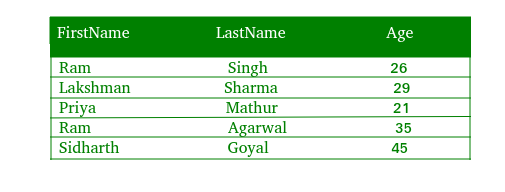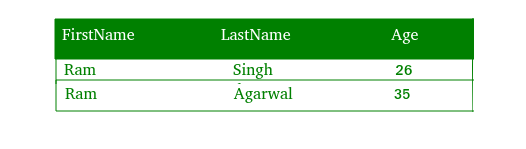GFG App
Open AppBrowser
Continue

The WHERE Clause is used to filter only those records that are fulfilled by a specific condition given by the user. in other words, the SQL WHERE clause is used to restrict the number of rows affected by a SELECT, UPDATE or DELETE query.

Syntax :
The basic syntax of the where clause is –
SELECT Column1 , Column2 , ….
FROM Table_Name
WHERE Condition

#### Implementation of WHERE Clause :

Let us consider the following table “Data” with three columns ‘FirstName’, ‘LastName’ and ‘Age’.To select all the rows where the “Firstname” is “ram”, we will use the following code :

Where Clause using Procedural Method :

 ` 0){ ` `        ``echo "<``table``>"; ` `            ``echo "<``tr``>"; ` `                ``echo "<``th``>Firstname"; ` `                ``echo "<``th``>Lastname"; ` `                ``echo "<``th``>age"; ` `            ``echo ""; ` `        ``while(\$row = mysqli_fetch_array(\$res)){ ` `            ``echo "<``tr``>"; ` `                ``echo "<``td``>" . \$row['Firstname'] . ""; ` `                ``echo "<``td``>" . \$row['Lastname'] . ""; ` `                ``echo "<``td``>" . \$row['Age'] . ""; ` `            ``echo ""; ` `        ``} ` `        ``echo ""; ` `        ``mysqli_free_result(\$res); ` `    ``} else{ ` `        ``echo "No Matching records are found."; ` `    ``} ` `} else{ ` `    ``echo "ERROR: Could not able to execute \$sql. "  ` `                                ``. mysqli_error(\$link); ` `} ` ` `  `mysqli_close(\$link); ` `?> `

Output :Code Explanation :

1. The “res” variable stores the data that is returned by the function mysql_query().
2. Everytime mysqli_fetch_array() is invoked, it returns the next row from the res() set.
3. The while loop is used to loop through all the rows of the table “data”.

Where Clause using Object Oriented Method :

 `connect_error); ` `} ` ` `  `\$sql = "SELECT * FROM Data WHERE Firstname='ram'"; ` `if(\$res = \$mysqli->query(\$sql)){ ` `    ``if(\$res->num_rows > 0){ ` `        ``echo "<``table``>"; ` `            ``echo "<``tr``>"; ` `                ``echo "<``th``>Firstname"; ` `                ``echo "<``th``>Lastname"; ` `                ``echo "<``th``>Age"; ` `            ``echo ""; ` `        ``while(\$row = \$res->fetch_array()){ ` `            ``echo "<``tr``>"; ` `                ``echo "<``td``>" . \$row['Firstname'] . ""; ` `                ``echo "<``td``>" . \$row['Lastname'] . ""; ` `                ``echo "<``td``>" . \$row['Age'] . ""; ` `            ``echo ""; ` `        ``} ` `        ``echo ""; ` `        ``\$res->free(); ` `    ``} else{ ` `        ``echo "No matching records are found."; ` `    ``} ` `} else{ ` `    ``echo "ERROR: Could not able to execute \$sql. "  ` `                                    ``. \$mysqli->error; ` `} ` ` `  `\$mysqli->close(); ` `?> `

Output :Where Clause using PDO Method :

 `setAttribute(PDO::ATTR_ERRMODE,  ` `                        ``PDO::ERRMODE_EXCEPTION); ` `} catch(PDOException \$e){ ` `    ``die("ERROR: Could not connect. "  ` `                    ``. \$e->getMessage()); ` `} ` ` `  `try{ ` `    ``\$sql = "SELECT * FROM Data WHERE Firstname='ram'";  ` `    ``\$res = \$pdo->query(\$sql); ` `    ``if(\$res->rowCount() > 0){ ` `        ``echo "<``table``>"; ` `            ``echo "<``tr``>"; ` `                ``echo "<``th``>Firstname"; ` `                ``echo "<``th``>Lastname"; ` `                ``echo "<``th``>Age"; ` `            ``echo ""; ` `        ``while(\$row = \$res->fetch()){ ` `            ``echo "<``tr``>"; ` `                ``echo "<``td``>" . \$row['Firstname'] . ""; ` `                ``echo "<``td``>" . \$row['Lastname'] . ""; ` `                ``echo "<``td``>" . \$row['Age'] . ""; ` `            ``echo ""; ` `        ``} ` `        ``echo ""; ` `        ``unset(\$res); ` `    ``} else{ ` `        ``echo "No records matching are found."; ` `    ``} ` `} catch(PDOException \$e){ ` `    ``die("ERROR: Could not able to execute \$sql. "  ` `                                   ``. \$e->getMessage()); ` `} ` ` `  `unset(\$pdo); ` `?> `

Output :My Personal Notes arrow_drop_up